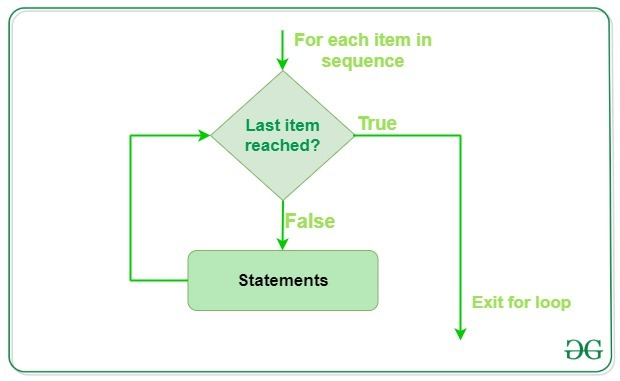# Python For Loops

For loops, in general, are used for sequential traversal. It falls under the category of definite iteration. Definite iterations means the number of repetitions is specified explicitly in advance.
Note: In python, for loops only implements the collection-based iteration.

## For in loops

For loops are used for sequential traversal. For example: traversing a list or string or array etc. In Python, there is no C style for loop, i.e., `for (i=0; i<n; i++)`. There is “for in” loop which is similar to for each loop in other languages. Let us learn how to use for in loop for sequential traversals.

Syntax:

```for var in iterable:
# statements
```

Here the `iterable `is a collection of objects like list, tuple. The indented statements inside the for loops are executed once for each item in an iterable. The variable var takes the value of next item of the iterable each time through the loop.

Example:

 `# Python program to illustrate  ` `# Iterating over a list  ` `print``(``"List Iteration"``)  ` `l ``=` `[``"geeks"``, ``"for"``, ``"geeks"``]  ` `for` `i ``in` `l:  ` `    ``print``(i)  ` `        `  `# Iterating over a tuple (immutable)  ` `print``(``"\nTuple Iteration"``)  ` `t ``=` `(``"geeks"``, ``"for"``, ``"geeks"``)  ` `for` `i ``in` `t:  ` `    ``print``(i)  ` `        `  `# Iterating over a String  ` `print``(``"\nString Iteration"``)      ` `s ``=` `"Geeks"` `for` `i ``in` `s :  ` `    ``print``(i)  ` `        `  `# Iterating over dictionary  ` `print``(``"\nDictionary Iteration"``)     ` `d ``=` `dict``()   ` `d[``'xyz'``] ``=` `123` `d[``'abc'``] ``=` `345` `for` `i ``in` `d :  ` `    ``print``(``"% s % d"` `%``(i, d[i]))  `

Output:

```List Iteration
geeks
for
geeks

Tuple Iteration
geeks
for
geeks

String Iteration
G
e
e
k
s

Dictionary Iteration
xyz 123
abc 345
```

Working of for loop :## Loop Control Statements

Loop control statements change execution from its normal sequence. When execution leaves a scope, all automatic objects that were created in that scope are destroyed. Python supports the following control statements.

• Continue Statement: It returns the control to the beginning of the loop.

 `# Prints all letters except 'e' and 's'  ` `for` `letter ``in` `'geeksforgeeks'``:  ` `    ``if` `letter ``=``=` `'e'` `or` `letter ``=``=` `'s'``:  ` `        ``continue` `    ``print``(``'Current Letter :'``, letter) ` `    ``var ``=` `10`

Output:

```Current Letter : g
Current Letter : k
Current Letter : f
Current Letter : o
Current Letter : r
Current Letter : g
Current Letter : k
```
• Break Statement: It brings control out of the loop.

 `for` `letter ``in` `'geeksforgeeks'``:   ` `   `  `    ``# break the loop as soon it sees 'e'   ` `    ``# or 's'  ` `    ``if` `letter ``=``=` `'e'` `or` `letter ``=``=` `'s'``:  ` `         ``break` `   `  `print``(``'Current Letter :'``, letter) `

Output:

```Current Letter : e
```
• Pass Statement: We use pass statement to write empty loops. Pass is also used for empty control statements, function and classes.

 `# An empty loop  ` `for` `letter ``in` `'geeksforgeeks'``:  ` `    ``pass` `print``(``'Last Letter :'``, letter) `

Output:

```Last Letter : s
```

## range() function

`range() `is a built-in function of Python. It is used when a user needs to perform an action for a specific number of times. `range()` in Python(3.x) is just a renamed version of a function called `xrange()` in Python(2.x). The range() function is used to generate a sequence of numbers.
In simple terms, `range()` allows user to generate a series of numbers within a given range. Depending on how many arguments user is passing to the function, user can decide where that series of numbers will begin and end as well as how big the difference will be between one number and the `next.range()` takes mainly three arguments.

• start: integer starting from which the sequence of integers is to be returned
• stop: integer before which the sequence of integers is to be returned.
The range of integers end at stop – 1.

• step: integer value which determines the increment between each integer in the sequence

 `# Python Program to  ` `# show range() basics  ` ` `  `# printing a number  ` `for` `i ``in` `range``(``10``):  ` `    ``print``(i, end ``=``" "``)  ` `print``()  ` ` `  `# using range for iteration  ` `l ``=` `[``10``, ``20``, ``30``, ``40``]  ` `for` `i ``in` `range``(``len``(l)):  ` `    ``print``(l[i], end ``=``" "``)  ` `print``()  ` ` `  `# performing sum of natural  ` `# number  ` `sum` `=` `0` `for` `i ``in` `range``(``1``, ``10``):  ` `    ``sum` `=` `sum` `+` `i  ` `print``(``"Sum of first 10 natural number :"``, ``sum``)  `

Output:

```0 1 2 3 4 5 6 7 8 9
10 20 30 40
Sum of first 10 natural number : 45
```

Note: To know more about `range()` click here.

## for-else loop

In most of the programming languages (C/C++, Java, etc), the use of else statement has been restricted with the if conditional statements. But Python also allows us to use the else condition with for loops.

Note: The else block just after for/while is executed only when the loop is NOT terminated by a break statement.

 `# Python program to demonstrate ` `# for-else loop ` ` `  `for` `i ``in` `range``(``1``, ``4``):  ` `    ``print``(i)  ` `else``: ``# Executed because no break in for  ` `    ``print``(``"No Break\n"``)  ` ` `  `for` `i ``in` `range``(``1``, ``4``):  ` `    ``print``(i)  ` `    ``break` `else``: ``# Not executed as there is a break  ` `    ``print``(``"No Break"``)  `

Output:

```1
2
3
No Break

1
```

Note: To know more about `for-else` loop click here.

My Personal Notes arrow_drop_upCheck out this Author's contributed articles.

If you like GeeksforGeeks and would like to contribute, you can also write an article using contribute.geeksforgeeks.org or mail your article to contribute@geeksforgeeks.org. See your article appearing on the GeeksforGeeks main page and help other Geeks.

Please Improve this article if you find anything incorrect by clicking on the "Improve Article" button below.

Article Tags :

2

Please write to us at contribute@geeksforgeeks.org to report any issue with the above content.